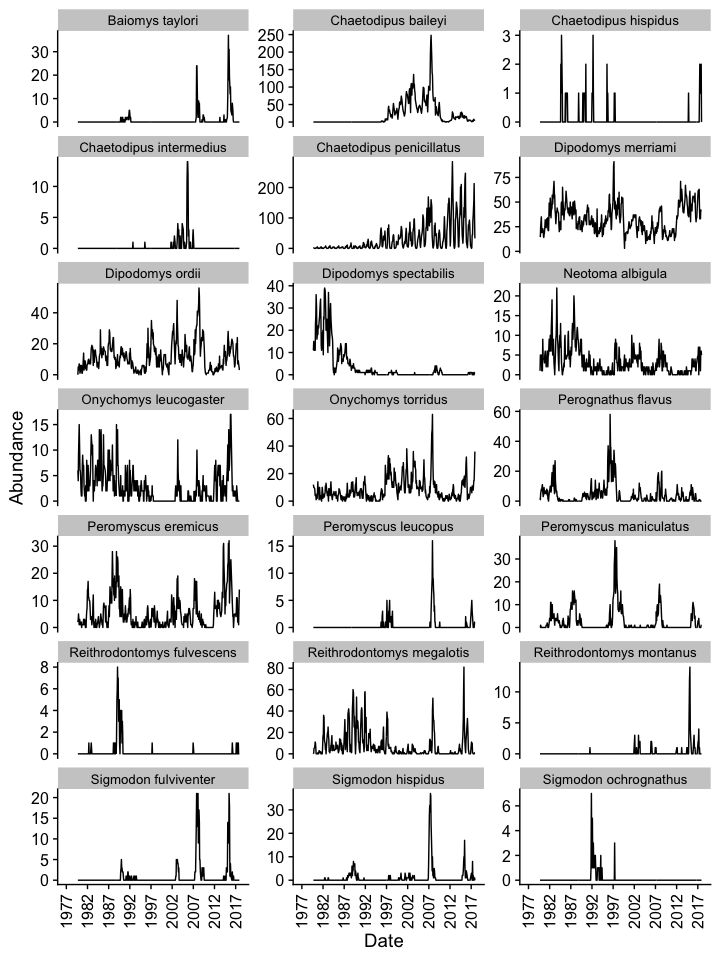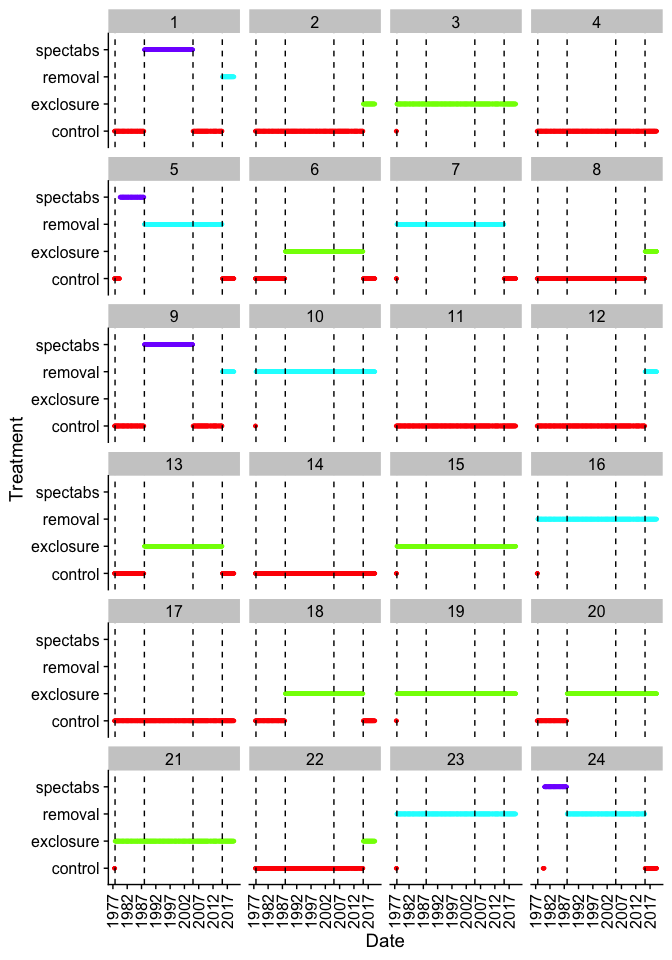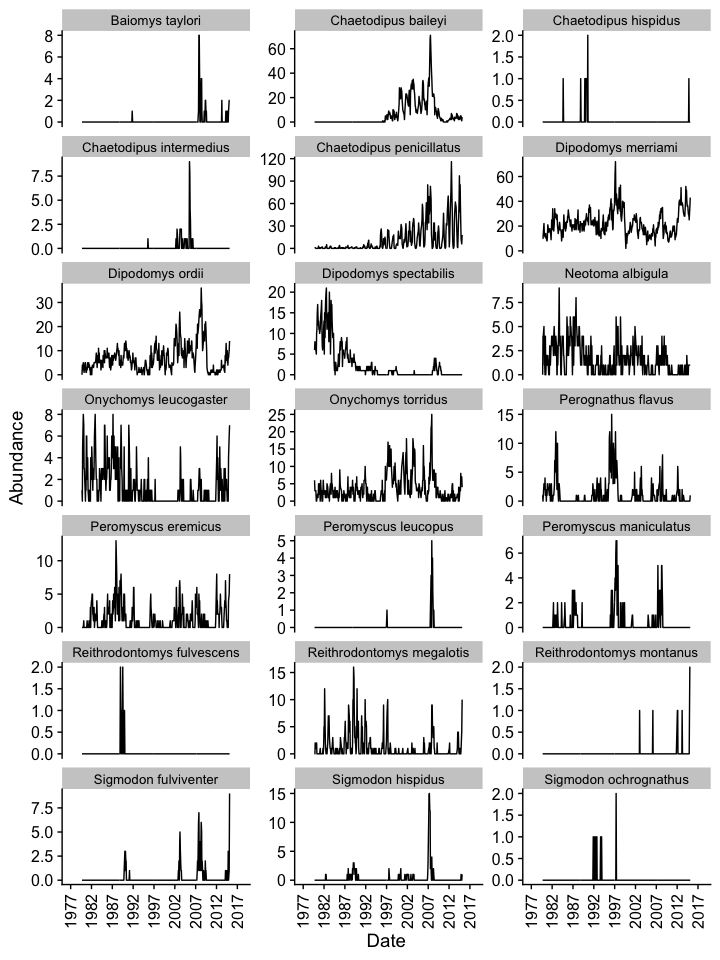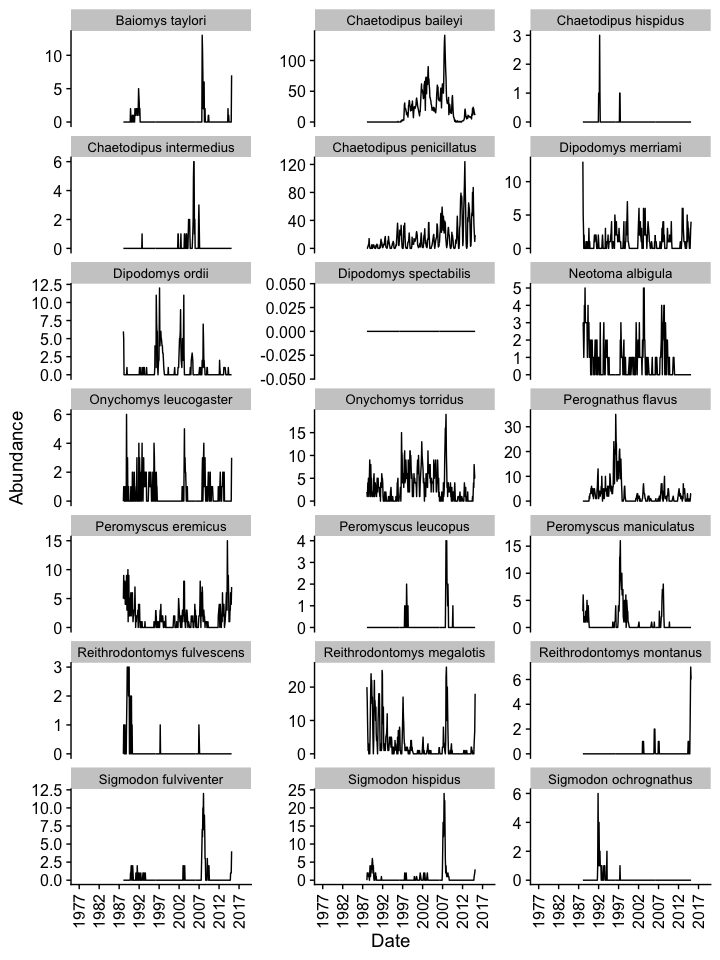# Introduction

This vignette is a basic guide to begin exploring the Portal data. We load in the data (making sure that we’re using the most recent copy from GitHub), and then explore the rodent abundances over time, with a comparison between the “control” and “kangaroo rat exclosure” treatments.

## Package Setup

• `tidyverse` contains several packages for data manipulation and plotting.

• `cowplot` contains a nicer default theme for ggplot.

• `portalr` is this package, which contains functions to read in the Portal data and do some basic processing.

``````library(dplyr)
library(tidyr)
library(ggplot2)
library(cowplot)
library(portalr)``````

# Retrieving the Data

Note that this package does not contain the actual Portal data, which resides online in a GitHub repository.

First, we try to load the data. If there isn’t a Portal folder, than `load_rodent_data` will fall back to downloading the data, as well.

check whether we already have the data. If we don’t have the data, or if the version we have isn’t the most recent, we use the `download_observations` function to download the latest copy of the data.

``````portal_data_path <- tempdir() # use a temporary folder to store downloaded data
#> ℹ Running gh query
#> ℹ Running gh query, got 100 records of about 300
#> ℹ Running gh query, got 300 records of about 450

The `load_rodent_data` function reads in several tables related to the rodent abundances. We won’t necessarily use all of these tables, but loading this now gives us access later.

``````print(summary(data_tables))
#>                Length Class      Mode
#> rodent_data    29     data.frame list
#> species_table  15     data.frame list
#> trapping_table  8     data.frame list
#> newmoons_table  4     data.frame list
#> plots_table     6     data.frame list``````

# Rodent Abundances

The first table that we loaded (`data_tables\$rodent_data`) is a record of whatever was found in the traps, mostly rodents, but also a few other taxa. If we just wanted to get the rodent abundance data, we could use the `abundance` function, which has default arguments to filter out the non-rodents.

``````# get rodent abundance by plot
rodent_abundance_by_plot <- abundance(path = portal_data_path, time = "date", level = "plot")

rodent_abundance <- rodent_abundance_by_plot %>%
gather(species, abundance, -censusdate, -treatment, -plot) %>%
count(species, censusdate, wt = abundance) %>%
rename(abundance = n)
print(summary(rodent_abundance))
#>    species            censusdate           abundance
#>  Length:8904        Min.   :1979-09-22   Min.   :  0.000
#>  Class :character   1st Qu.:1989-04-29   1st Qu.:  0.000
#>  Mode  :character   Median :1998-02-14   Median :  0.000
#>                     Mean   :1998-12-05   Mean   :  6.695
#>                     3rd Qu.:2008-10-04   3rd Qu.:  5.000
#>                     Max.   :2019-05-04   Max.   :285.000``````

Let’s convert the data to long format for easier facetting. Also, we want the scientific names instead of the two-letter species codes, so let’s do that matching, too:

``````join_scientific_name <- function(rodent_abundance,
species_table = data_tables\$species_table)
{
return(rodent_abundance %>%
left_join(select(species_table, "species", "scientificname"),
by = "species") %>%
rename(scientific_name = scientificname)
)
}

rodent_abundance <- join_scientific_name(rodent_abundance)``````

## Figure: abundance over time

``````make_abundance_plot_over_time <- function(rodent_abundance)
{
return(ggplot(rodent_abundance,
aes(x = censusdate, y = abundance)) +
geom_line() +
facet_wrap(~scientific_name, scales = "free_y", ncol = 3) +
xlab("Date") +
ylab("Abundance") +
scale_x_date(breaks = seq(as.Date("1977-01-01"), to = as.Date("2018-01-01"), "+5 years"),
date_labels = "%Y",
limits = as.Date(c("1977-01-01", "2018-01-01"))) +
theme_cowplot() +
theme(axis.text.x = element_text(angle = 90, hjust = 1, vjust = 0.5),
legend.position = "bottom", legend.justification = "center",
strip.text.x = element_text(size = 10))
)
}

my_plot <- make_abundance_plot_over_time(rodent_abundance)

print(my_plot)
#> Warning: Removed 12 row(s) containing missing values (geom_path).``````Monthly abundance of rodents (all plots)

## Next Steps

Our next steps would likely be to dig deeper into the rodent abundances for different treatments, but first we want to know what the different treatments look like, so let’s revisit the abundances later.

# Plot Treatments

A description of the experimental design and treatments can be found in this Readme file in the PortalDate repo.

For now, we are just going to use the `Portal_plots` table file to look at how the treatments for individual plots have changed over time. Note that this file is already loaded in as the `plots_table` from the `loadData` function we ran previously.

``````print(summary(data_tables\$plots_table))
#>       year          month             plot        treatment
#>  Min.   :1977   Min.   : 1.000   Min.   : 1.00   Length:10856
#>  1st Qu.:1988   1st Qu.: 4.000   1st Qu.: 6.00   Class :character
#>  Median :1998   Median : 7.000   Median :12.00   Mode  :character
#>  Mean   :1998   Mean   : 6.525   Mean   :12.49
#>  3rd Qu.:2008   3rd Qu.: 9.000   3rd Qu.:18.00
#>  Max.   :2019   Max.   :12.000   Max.   :24.00
#>  resourcetreatment  anttreatment
#>  Length:10856       Length:10856
#>  Class :character   Class :character
#>  Mode  :character   Mode  :character
#>
#>
#> ``````

We want a proper date variable as well as converting `plot` into a factor:

``````plot_treatments <- data_tables\$plots_table %>%
mutate(iso_date = as.Date(paste0(year, "-", month, "-", "01")),
plot = as.factor(plot)) %>%
select(iso_date, plot, treatment)``````

## Figure: plot treatments over time

``````my_plot <- ggplot(plot_treatments,
aes(x = iso_date, y = treatment, color = treatment)) +
geom_point(shape = 20) +
geom_vline(aes(xintercept = as.Date("1977-10-01")), linetype = 2) +
geom_vline(aes(xintercept = as.Date("1988-01-01")), linetype = 2) +
geom_vline(aes(xintercept = as.Date("2005-01-01")), linetype = 2) +
geom_vline(aes(xintercept = as.Date("2015-04-01")), linetype = 2) +
facet_wrap(~plot, ncol = 4) +
xlab("Date") +
ylab("Treatment") +
scale_color_manual(values = rainbow(4)) +
scale_x_date(breaks = seq(as.Date("1977-01-01"), to = as.Date("2018-01-01"), "+5 years"), date_labels = "%Y") +
theme_cowplot() +
guides(color = FALSE) +
theme(axis.text.x = element_text(angle = 90, hjust = 1, vjust = 0.5))

print(my_plot)``````Treatments of Plots by Date

## Identifying control plots

The treatments for the plots have changed over time: in some cases, this was due to initial ramping up of the experimental protocol, in others, exclusions of Dipodomys spectabilis were started and then converted back later because the species went locally extinct (e.g. plots 1, 5, 9, 24).

### Which plots have always been control plots?

``````always_control_plots <- plot_treatments %>%
group_by(plot) %>%
summarize(always_control = all(treatment == "control")) %>%
filter(always_control)

print(always_control_plots)
#> # A tibble: 4 x 2
#>   plot  always_control
#>   <fct> <lgl>
#> 1 4     TRUE
#> 2 11    TRUE
#> 3 14    TRUE
#> 4 17    TRUE``````

Note, however, that this excludes several plots for which the treatment was changed ~2015. We can include these plots by first filtering by date before testing for the “control” treatment:

``````mostly_control_plots <- plot_treatments %>%
filter(iso_date < "2015-01-01") %>%
group_by(plot) %>%
summarize(mostly_control = all(treatment == "control")) %>%
filter(mostly_control)

print(mostly_control_plots)
#> # A tibble: 8 x 2
#>   plot  mostly_control
#>   <fct> <lgl>
#> 1 2     TRUE
#> 2 4     TRUE
#> 3 8     TRUE
#> 4 11    TRUE
#> 5 12    TRUE
#> 6 14    TRUE
#> 7 17    TRUE
#> 8 22    TRUE``````

And to identify the datespan over which these plots have been controls:

``````date_span <- plot_treatments %>%
filter(plot %in% mostly_control_plots\$plot) %>%
group_by(iso_date) %>%
summarize(all_control = all(treatment == "control")) %>%
filter(all_control)

print(date_span)
#> # A tibble: 411 x 2
#>    iso_date   all_control
#>    <date>     <lgl>
#>  1 1977-07-01 TRUE
#>  2 1977-08-01 TRUE
#>  3 1977-09-01 TRUE
#>  4 1977-10-01 TRUE
#>  5 1977-11-01 TRUE
#>  6 1977-12-01 TRUE
#>  7 1978-01-01 TRUE
#>  8 1978-02-01 TRUE
#>  9 1978-03-01 TRUE
#> 10 1978-04-01 TRUE
#> # … with 401 more rows``````

## Abundances over control plots

We are now ready to plot abundances just over the control plots and the time span in 1977-07-01 to 2015-03-01. We do this by retrieving the abundance data by plot, and then filtering accordingly:

``````rodent_abundance_by_plot %>%
filter(censusdate >= min(date_span\$iso_date),
censusdate <= max(date_span\$iso_date),
plot %in% mostly_control_plots\$plot) %>%
select(-treatment, -plot) %>%
gather(species, abundance, -censusdate) %>%
count(censusdate, species, wt = abundance) %>%
rename(abundance = n) %>%
join_scientific_name() %>%
{.} -> rodent_abundance_control

rodent_abundance_control %>%
make_abundance_plot_over_time() %>%
print()``````Monthly abundance of rodents (control plots)

## Abundances over rodent exclosures

We can do the same with the “exclosure” condition. First, which plots:

``````mostly_exclosure_plots <- plot_treatments %>%
filter(iso_date > as.Date("1989-01-01"),
iso_date < "2015-01-01") %>%
group_by(plot) %>%
summarize(mostly_exclosure = all(treatment == "exclosure")) %>%
filter(mostly_exclosure)

print(mostly_exclosure_plots)
#> # A tibble: 8 x 2
#>   plot  mostly_exclosure
#>   <fct> <lgl>
#> 1 3     TRUE
#> 2 6     TRUE
#> 3 13    TRUE
#> 4 15    TRUE
#> 5 18    TRUE
#> 6 19    TRUE
#> 7 20    TRUE
#> 8 21    TRUE``````

Then, the datespan:

``````date_span <- plot_treatments %>%
filter(plot %in% mostly_exclosure_plots\$plot) %>%
group_by(iso_date) %>%
summarize(all_exclosure = all(treatment == "exclosure")) %>%
filter(all_exclosure)

print(date_span)
#> # A tibble: 297 x 2
#>    iso_date   all_exclosure
#>    <date>     <lgl>
#>  1 1988-01-01 TRUE
#>  2 1988-02-01 TRUE
#>  3 1988-03-01 TRUE
#>  4 1988-04-01 TRUE
#>  5 1988-05-01 TRUE
#>  6 1988-06-01 TRUE
#>  7 1988-07-01 TRUE
#>  8 1988-08-01 TRUE
#>  9 1988-09-01 TRUE
#> 10 1988-10-01 TRUE
#> # … with 287 more rows``````

Finally, the figure:

``````rodent_abundance_by_plot %>%
filter(censusdate >= min(date_span\$iso_date),
censusdate <= max(date_span\$iso_date),
plot %in% mostly_exclosure_plots\$plot) %>%
select(-treatment, -plot) %>%
gather(species, abundance, -censusdate) %>%
count(censusdate, species, wt = abundance) %>%
rename(abundance = n) %>%
join_scientific_name() %>%
{.} -> rodent_abundance_exclosure

rodent_abundance_exclosure %>%
make_abundance_plot_over_time() %>%
print()``````Monthly abundance of rodents (exclosure plots)

Since these data have the same number of plots as the previous figure, we can directly compare abundances. Note the decreased numbers for kangaroo rats (Dipodomys spp.) and increased numbers for some other taxa.

## Comparison Figure

Let’s merge the two datasets and produce a combined plot:

``````rodent_abundance_merged <- bind_rows(
mutate(rodent_abundance_control, treatment = "control"),
mutate(rodent_abundance_exclosure, treatment = "exclosure"))

merged_plot <- ggplot(rodent_abundance_merged,
aes(x = censusdate, y = abundance, color = treatment)) +
geom_line() +
facet_wrap(~scientific_name, scales = "free_y", ncol = 3) +
xlab("Date") +
ylab("Abundance") +
scale_x_date(breaks = seq(as.Date("1977-01-01"), to = as.Date("2018-01-01"), "+5 years"),
date_labels = "%Y",
limits = as.Date(c("1977-01-01", "2018-01-01"))) +
scale_color_manual(values = c("purple", "yellow")) +
theme_cowplot() +
theme(axis.text.x = element_text(angle = 90, hjust = 1, vjust = 0.5),
legend.position = "bottom", legend.justification = "center",
strip.text.x = element_text(size = 10))

print(merged_plot)``````